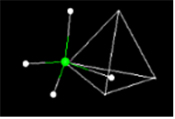## MathMol Textbook Version3 (Beta)

Part 1 - Introduction to molecular modeling and simple software, Part 2 contains Flash animation of a molecular dynamics simulation of a fatty acid protein.

Why is Molecular Modeling Important? Designing drugs for the future, designing new materials, view a bukeytube in 3-D

What do Some Common Molecules Look Like?View in 3-D: water, methane, benzene, aspirin, DNA..

Where's the Math? Part1: Geometry, symmetry; Part 2: Mathematical Relationships; Part 3: Overview of MathMol Tutorials..

Carbon 3 WaysView in 3-D graphite, diamond, buckyball, buckytube.

How to View Molecular Structures in Class or at Home Selection of free software that can be used at home or in class

MathMol Library of Structures. Water and Ice, Carbon, Hydrocarbons, Molecules of Life, Drugs

Activity1: Measuring length and distance at the molecular level When we measure length or distance at the molecular level we are talking of a very small measurement. This activity introduces you to the software Jsmol and is used to measure line segments at the size of molecules.

Activity2: Geometry-of-1-Dimension In this lesson we begin to take a look at the 3-Dimensions of the world we live in. In this activity we look at the geometry of 1-Dimension.

Activity3: Geometry of 2- Dimensions When we see a graph on a page we really don't think in terms of dimensions. But the graph above has only two axis or two dimensions. It is a plane. ... see Geometry of 2-Dimensions

Activity4: Geometry of 3-Dimensions Molecules are solid 3 dimensional objects in 3 dimensional space. To describe molecules accurately we must describe their postion in 3-D space.

Activity5: Introduction to Molecular Modeling using Jsmol Using Jsmol you will view simple molecules -- water, methane, aspirin. Hydrocarbons Methane to Octane....

Activity6: The Geometry of Crystals ----diamond --- ice --salt calcium chloride... see also ---https://www.worldofmolecules.com/3D/why-does-salt-have-a-cubic-crystal-structure.html

Activity7: Summary Sheet by Students This is a summary sheet to test the students knowledge of previous activities...

Activity8: What is the Geometry of the Methane Molecule We look at the geometry of the methane molecule in this interactive activity.

Activity9: Geometry of the Crystal Structure of Ice

Why does ice float... we look at the unique crystal structure of ice crystals.

Activity10: Geometry of the Benzene Molecule

The benzene molecule has a common geometric shape as does cyclohexane. You will measure angles and learn about the bonds in the benzene molecule.

Appendix1: Scientific Notation Learn to convert standard notation to scientific notation and vica-versa..

Appendix 2: Mass Measure the mass of an unknown using a triple-beam balance.

Appendix3: Volume Measure the volume of an unkown using geometric liquid displacement methods...

Appendix4: Density Find the density of an unknown mass from it's mass and volume..

#### MATHMOLK-12 STEM INTERACTIVE ACTIVITIES